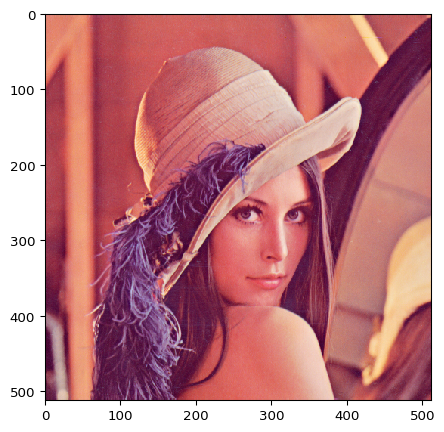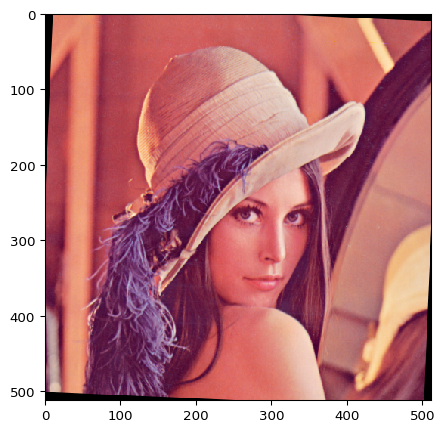# Load and Augment an Image¶

Expected input data. Augmenting an image with imgaug takes only a few lines of code. But before doing that, we first have to load the image. imgaug expects images to be numpy arrays and works best with dtype uint8, i.e. when the array's values are in the range 0 to 255. The channel-axis is always expected to be the last axis and may be skipped for grayscale images. For non-grayscale images, the expected input colorspace is RGB.

Non-uint8 data. If you work with other dtypes than uint8, such as float32, it is recommended to take a look at the dtype documentation for a rough overview of each augmenter's dtype support. The API contains further details. Keep in mind that uint8 is always the most thoroughly tested dtype.

Image loading function. As imgaug only deals with augmentation and not image input/output, we will need another library to load our image. A common choice to do that in python is imageio, which we will use below. Another common choice is OpenCV via its function cv2.imread(). Note however that cv2.imread() returns images in BGR colorspace and not RGB, which means that you will have to re-order the channel axis, e.g. via cv2.imread(path)[:, :, ::-1]. You could alternatively also change every colorspace-dependent augmenter to BGR (e.g. Grayscale or any augmenter changing hue and/or saturation). See the API for details per augmenter. The disadvantage of the latter method is that all visualization functions (such as imgaug.imshow() below) are still going to expect RGB data and hence BGR images will look broken.

## Load and Show an Image¶

Lets jump to our first example. We will use imageio.imread() to load an image and augment it. In the code block below, we call imageio.imread(uri) to load an image directly from wikipedia, but we could also load it from a filepath, e.g. via imagio.imread("/path/to/the/file.jpg") or for Windows imagio.imread("C:\\path\to\the\file.jpg"). imageio.imread(uri) returns a numpy array of dtype uint8, shape (height, width, channels) and RGB colorspace. That is exactly what we need. After loading the image, we use imgaug.imshow(array) to visualize the loaded image.

In :
import imageio
import imgaug as ia
%matplotlib inline

print("Original:")
ia.imshow(image)

Original:## Augment the Image¶

Now that we have loaded the image, let's augment it. imgaug contains many augmentation techniques in the form of classes deriving from the Augmenter parent class. To use one augmentation technique, we have to instantiate it with a set of hyperparameters and then later on apply it many times. Our first augmentation technique will be Affine, i.e. affine transformations. We keep it simple here and use that technique to rotate the image by a random value between -25° and +25°.

In :
from imgaug import augmenters as iaa
ia.seed(4)

rotate = iaa.Affine(rotate=(-25, 25))
image_aug = rotate(image=image)

print("Augmented:")
ia.imshow(image_aug)

Augmented:## Augment a Batch of Images¶

Of course, in reality we rarely just want to augment a single image. We can achieve this using the same code as above, just changing the signular parameter image to images. It is often significantly faster to augment a batch of images than to augment each image individually.

For simplicity, we create a batch here by just copying the original image several times and then feeding it through our rotation augmenter. To visualize our results, we use numpy's hstack() function, which combines the images in our augmented batch to one large image by placing them horizontally next to each other.

In :
import numpy as np

images = [image, image, image, image]
images_aug = rotate(images=images)

print("Augmented batch:")
ia.imshow(np.hstack(images_aug))

Augmented batch: